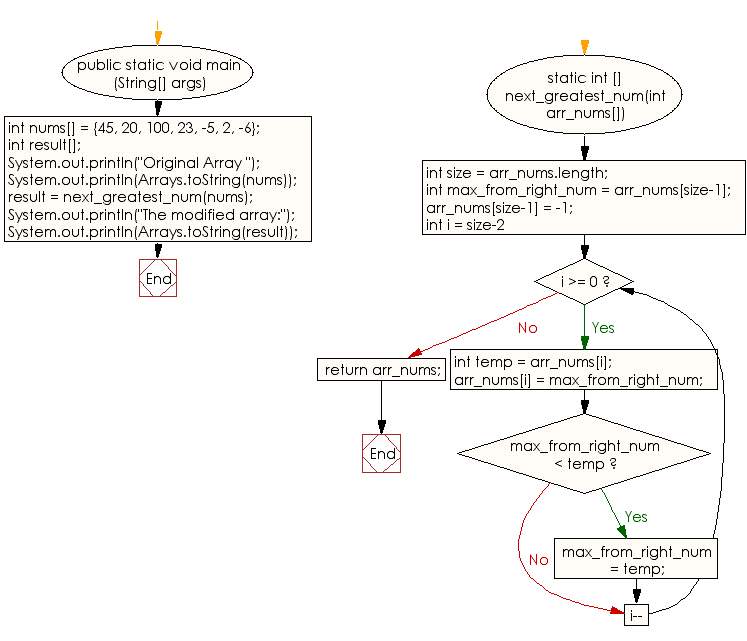﻿ Java: Elements of array is replaced with the next largest# Java Array Exercises: Replace every element with the next greatest element in a given array of integers

## Java Array: Exercise-53 with Solution

Write a Java program to replace every element with the next greatest element (from right side) in a given array of integers. There is no element next to the last element, therefore replace it with -1.

Sample Solution:

Java Code:

``````import java.io.*;
import java.util.Arrays;
public class Main
{
public static void main (String[] args)
{
int nums[] = {45, 20, 100, 23, -5, 2, -6};
int result[];
System.out.println("Original Array ");
System.out.println(Arrays.toString(nums));

result = next_greatest_num(nums);
System.out.println("The modified array:");
System.out.println(Arrays.toString(result));
}

static int [] next_greatest_num(int arr_nums[])
{
int size = arr_nums.length;
int max_from_right_num =  arr_nums[size-1];
arr_nums[size-1] = -1;

for (int i = size-2; i >= 0; i--)
{
int temp = arr_nums[i];
arr_nums[i] = max_from_right_num;
if(max_from_right_num < temp)
max_from_right_num = temp;
}
return arr_nums;
}
}
```
```

Sample Output:

```
Original Array
[45, 20, 100, 23, -5, 2, -6]
The modified array:
[100, 100, 23, 2, 2, -6, -1]
```

Flowchart:Visualize Java code execution (Python Tutor):

Java Code Editor:

Improve this sample solution and post your code through Disqus

What is the difficulty level of this exercise?

Test your Programming skills with w3resource's quiz.

﻿

## Java: Tips of the Day

getEnumMap

Converts to enum to Map where key is the name and value is Enum itself.

```public static <E extends Enum<E>> Map<String, E> getEnumMap(final Class<E> enumClass) {
return Arrays.stream(enumClass.getEnumConstants())
.collect(Collectors.toMap(Enum::name, Function.identity()));
}
```

Ref: https://bit.ly/3xXcFZt